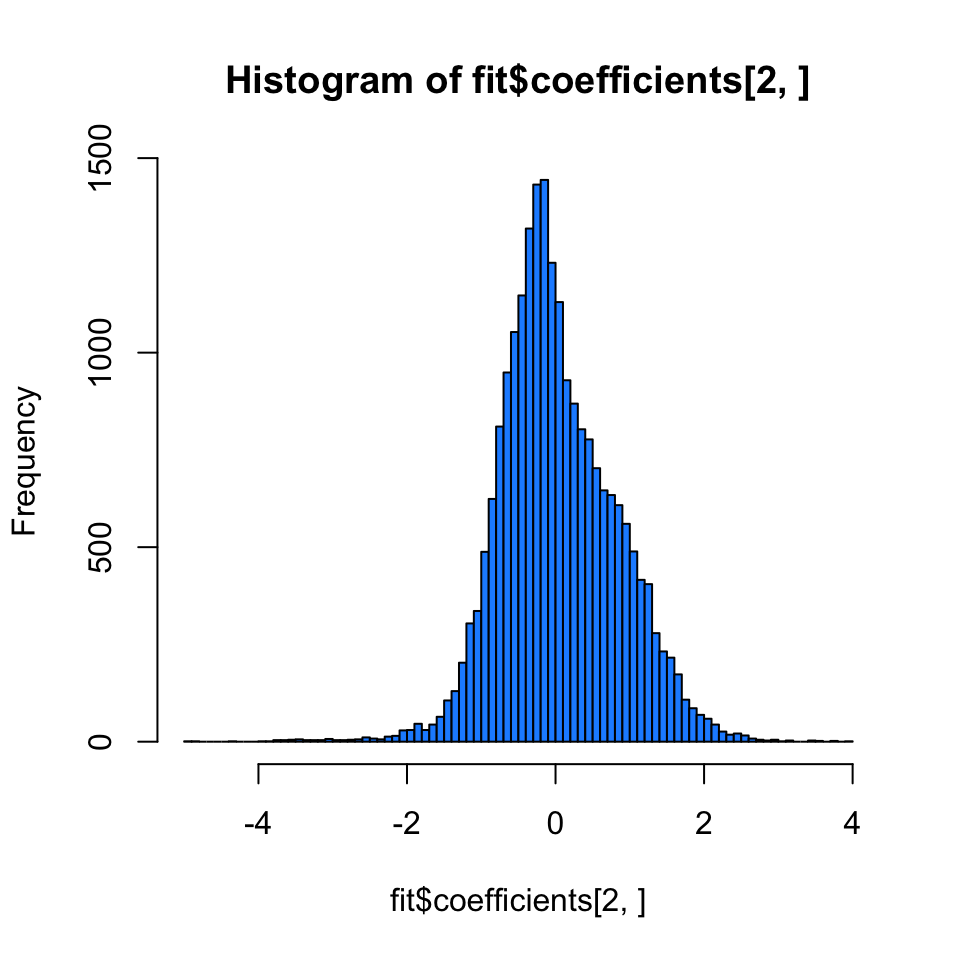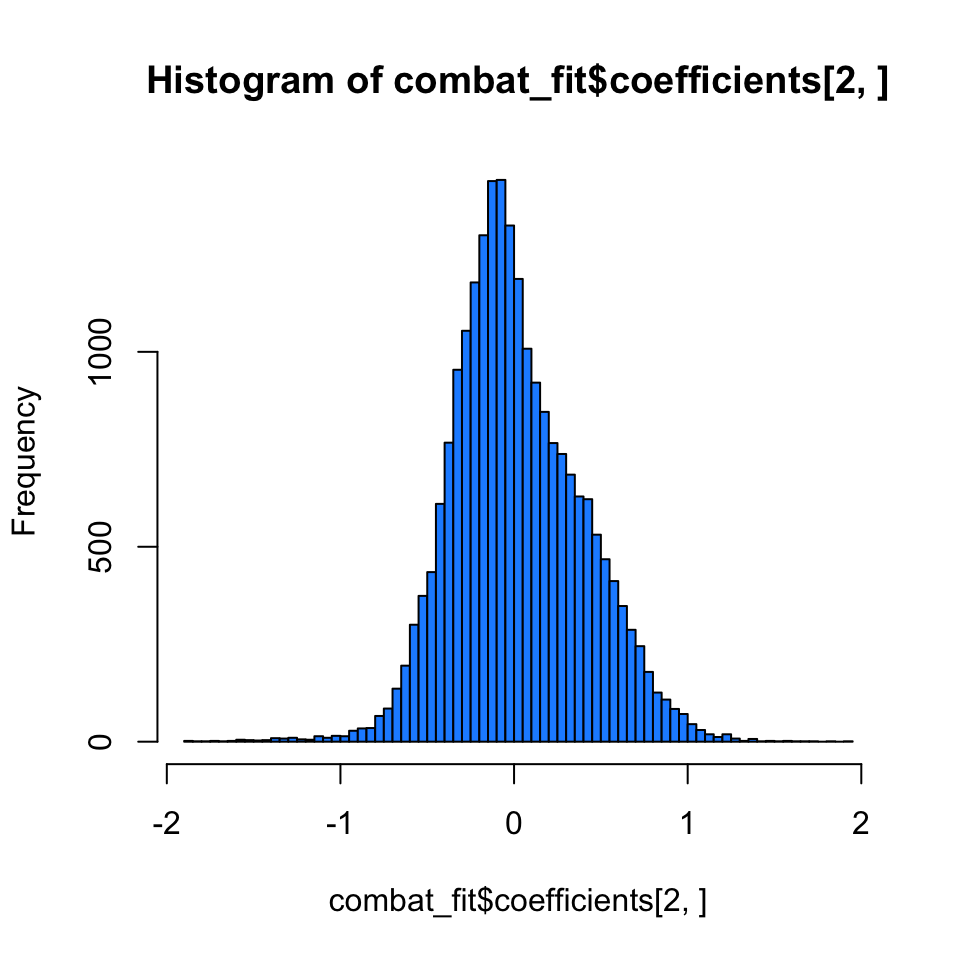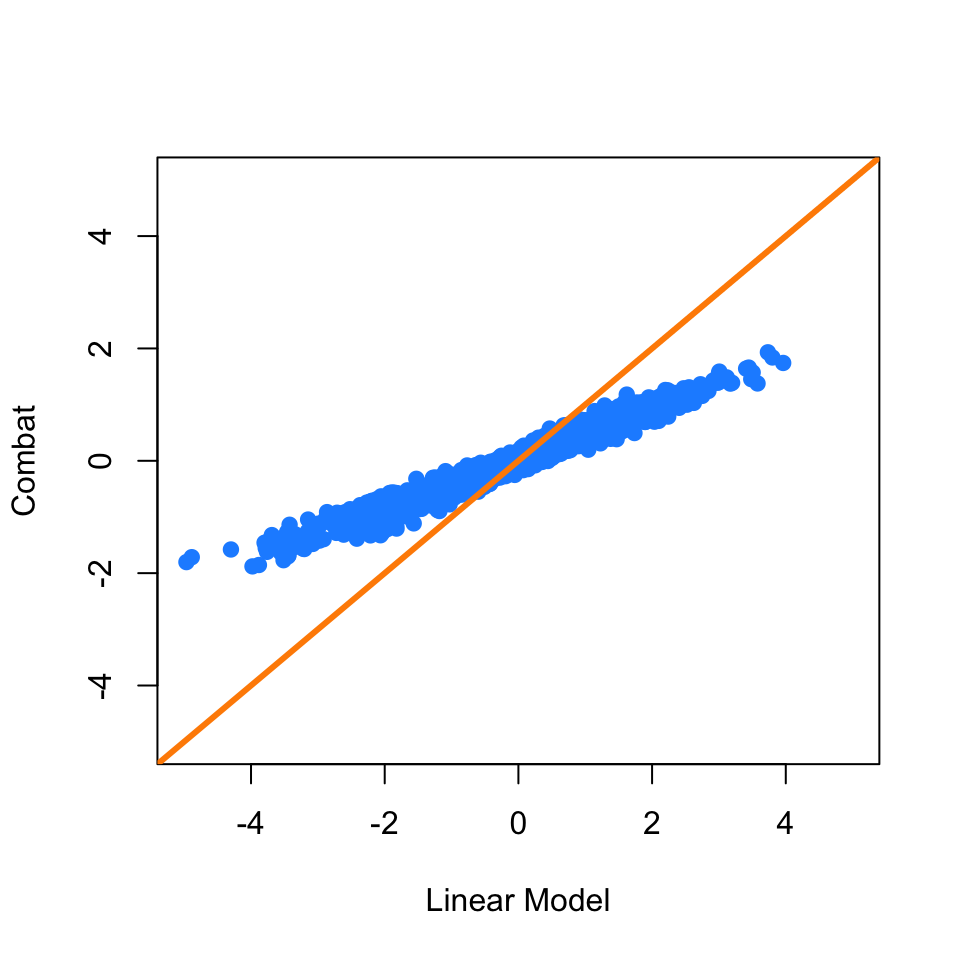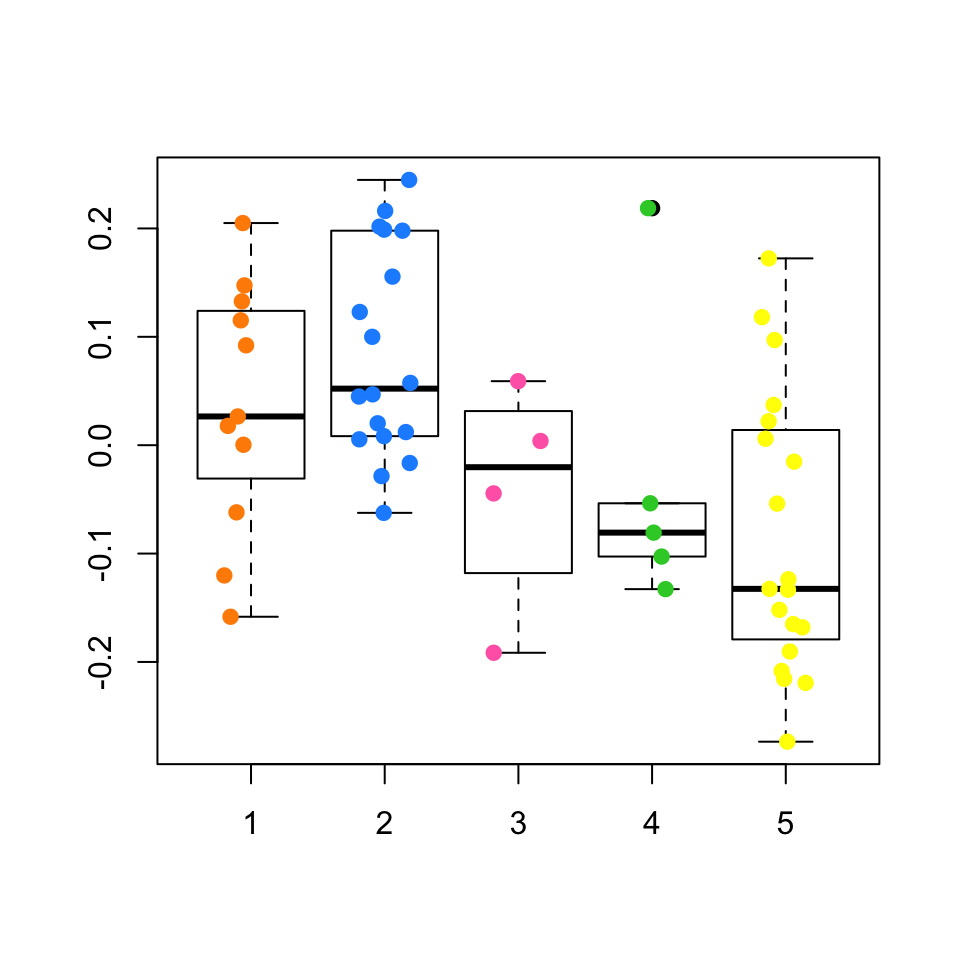## Dependencies

This document depends on the following packages:

``````  library(devtools)
library(Biobase)
library(sva)
library(snpStats)``````

To install these packages you can use the code (or if you are compiling the document, remove the `eval=FALSE` from the chunk.)

``````install.packages(c("devtools"))
source("http://www.bioconductor.org/biocLite.R")

The analyses performed in this experiment are based on gene expression measurements from a bladder cancer study: Gene expression in the urinary bladder: a common carcinoma in situ gene expression signature exists disregarding histopathological classification. The data can be loaded from the bladderbatch data package.

``data(bladderdata)``

## Set up the data

``````pheno = pData(bladderEset)

## Adjusting for batch effects with a linear model

We will use two models. One with the variable we care about (cancer status) and the other that is just the known adjustment variables (in this case we will assume none)

``````mod = model.matrix(~as.factor(cancer) + as.factor(batch),data=pheno)
fit = lm.fit(mod,t(edata))
hist(fit\$coefficients[2,],col=2,breaks=100)``````This will only work if the batch effects aren’t too highly correlated with the outcome. Let’s check that

``table(pheno\$cancer,pheno\$batch)``
``````##
##           1  2  3  4  5
##   Biopsy  0  0  0  5  4
##   Cancer 11 14  0  0 15
##   Normal  0  4  4  0  0``````

## Adjusting for batch effects with Combat

Another approach is to use Combat. Combat returns a “cleaned” data matrix after batch effects have been removed. Here we pass a model matrix with any known adjustment variables and a second parameter that is the batch variable.

``````batch = pheno\$batch
modcombat = model.matrix(~1, data=pheno)
modcancer = model.matrix(~cancer, data=pheno)
combat_edata = ComBat(dat=edata, batch=batch, mod=modcombat, par.prior=TRUE, prior.plots=FALSE)``````
``````## Found 5 batches
## Adjusting for 0 covariate(s) or covariate level(s)
## Standardizing Data across genes
## Fitting L/S model and finding priors
``````combat_fit = lm.fit(modcancer,t(combat_edata))
hist(combat_fit\$coefficients[2,],col=2,breaks=100)``````## Comparing Combat and linear adjustment

We can compare the estimated coefficients from Combat and linear adjustment by looking at the right coefficients for each model.

``````plot(fit\$coefficients[2,],combat_fit\$coefficients[2,],col=2,
xlab="Linear Model",ylab="Combat",xlim=c(-5,5),ylim=c(-5,5))
abline(c(0,1),col=1,lwd=3)``````## Adjusting for batch effects with sva

First we need to estimate the surrogate variables. To do this, we need to build a model with any known adjustment variables and the variable we care about `mod` and another model with only the adjustment variables. Here we won’t adjust for anything to see if `sva` can “discover” the batch effect.

``````mod = model.matrix(~cancer,data=pheno)
mod0 = model.matrix(~1, data=pheno)
sva1 = sva(edata,mod,mod0,n.sv=2)``````
``````## Number of significant surrogate variables is:  2
## Iteration (out of 5 ):1  2  3  4  5``````

See if any of the variables correlate with batch

``summary(lm(sva1\$sv ~ pheno\$batch))``
``````## Response Y1 :
##
## Call:
## lm(formula = Y1 ~ pheno\$batch)
##
## Residuals:
##      Min       1Q   Median       3Q      Max
## -0.26953 -0.11076  0.00787  0.10399  0.19069
##
## Coefficients:
##              Estimate Std. Error t value Pr(>|t|)
## (Intercept) -0.018470   0.038694  -0.477    0.635
## pheno\$batch  0.006051   0.011253   0.538    0.593
##
## Residual standard error: 0.1345 on 55 degrees of freedom
## Multiple R-squared:  0.00523,    Adjusted R-squared:  -0.01286
## F-statistic: 0.2891 on 1 and 55 DF,  p-value: 0.5929
##
##
## Response Y2 :
##
## Call:
## lm(formula = Y2 ~ pheno\$batch)
##
## Residuals:
##      Min       1Q   Median       3Q      Max
## -0.23973 -0.07467 -0.02157  0.08116  0.25629
##
## Coefficients:
##              Estimate Std. Error t value Pr(>|t|)
## (Intercept)  0.121112   0.034157   3.546 0.000808 ***
## pheno\$batch -0.039675   0.009933  -3.994 0.000194 ***
## ---
## Signif. codes:  0 '***' 0.001 '**' 0.01 '*' 0.05 '.' 0.1 ' ' 1
##
## Residual standard error: 0.1187 on 55 degrees of freedom
## Multiple R-squared:  0.2248, Adjusted R-squared:  0.2107
## F-statistic: 15.95 on 1 and 55 DF,  p-value: 0.0001945``````
``````boxplot(sva1\$sv[,2] ~ pheno\$batch)
points(sva1\$sv[,2] ~ jitter(as.numeric(pheno\$batch)),col=as.numeric(pheno\$batch))``````Add the surrogate variables to the model matrix and perform the model fit

``````modsv = cbind(mod,sva1\$sv)
fitsv = lm.fit(modsv,t(edata))``````

Compare the fit from surrogate variable analysis to the other two.

``````par(mfrow=c(1,2))
plot(fitsv\$coefficients[2,],combat_fit\$coefficients[2,],col=2,
xlab="SVA",ylab="Combat",xlim=c(-5,5),ylim=c(-5,5))
abline(c(0,1),col=1,lwd=3)
plot(fitsv\$coefficients[2,], fit\$coefficients[2,],col=2,
xlab="SVA",ylab="linear model",xlim=c(-5,5),ylim=c(-5,5))
abline(c(0,1),col=1,lwd=3)``````